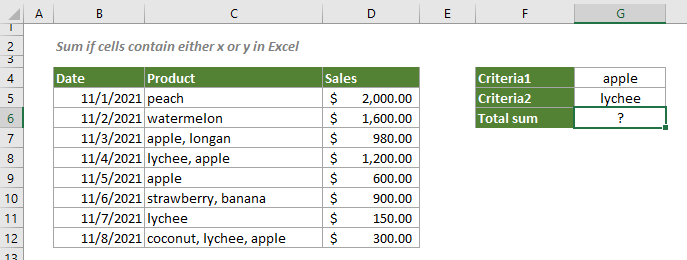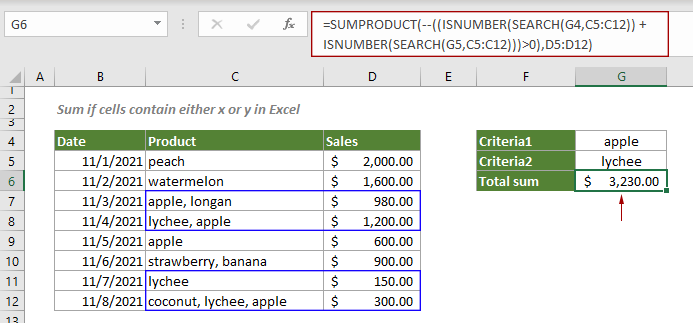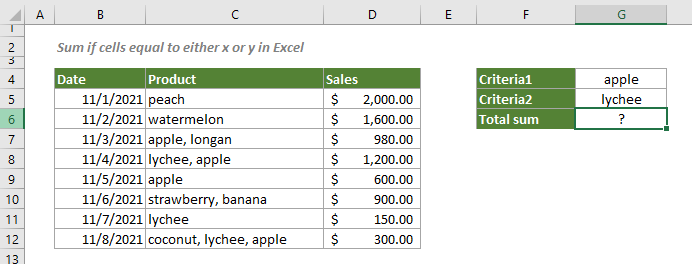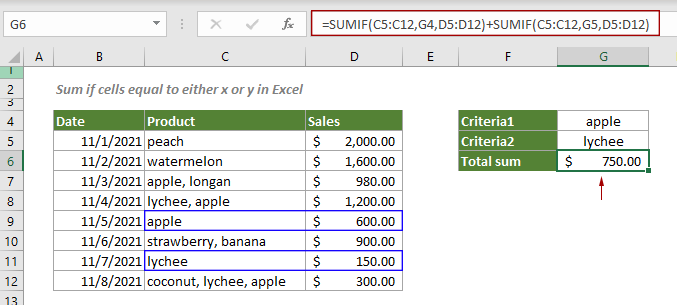## Sum if cells contain or equal to either x or y in Excel

To sum the cells if contain or equal to either one value or another, this tutorial provides two formulas in detail to help solve the problems.

How to sum if cells contain either x or y in Excel?
How to sum if cells equal to either x or y in Excel?

#### How to sum if cells contain either x or y in Excel?

As shown in the screenshot below, to sum the cells in column D if the cells in column C contain either “apple” or “lychee”, you can apply a formula based on the SUMPRODUCT, ISNUMBER and SEARCH functions.Generic Formulas

=SUMPRODUCT(--((ISNUMBER(SEARCH("criteria1",rng1)) + ISNUMBER(SEARCH("criteria2",rng2)))>0),sum_rng)

Arguments

Criteria_range1: The range of cells that you want evaluated by the criteria1;
Criteria_rang2: The range of cells that you want evaluated by the criteria2;
Criteria1: The criteria used to define which cells in Criteria_rang1 will be added;
Criteria2: The criteria used to define which cells in Criteria_rang2 will be added.
Sum_range: The range of cells to sum;

How to use this formula?

Select a blank cell, copy or enter the formula below and press the Enter key to get the result.

=SUMPRODUCT(--((ISNUMBER(SEARCH(G4,C5:C12))+ISNUMBER(SEARCH(G5,C5:C12)))>0),D5:D12)Notes: In this formula, G4 and G5 are the cells containing the two conditions. Alternatively, you can directly type in the actual text values that enclosed in double quotation marks.

=SUMPRODUCT(--((ISNUMBER(SEARCH("apple",C5:C12))+ISNUMBER(SEARCH("lychee",C5:C12)))>0),D5:D12)

Explanation of the formula

=SUMPRODUCT(--((ISNUMBER(SEARCH("apple",C5:C12))+ISNUMBER(SEARCH("lychee",C5:C12)))>0),D5:D12)

ISNUMBER(SEARCH("apple",C5:C12))
> SEARCH("apple",C5:C12): The SEARCH function locates the position of the first character of “apple” in each cell of the range C5:C12, and returns an array like this {#VALUE!;#VALUE!;1;9;1;#VALUE!;#VALUE!;18}. The #VALUE!’s mean that “apple” is not found in the corresponding cell;
> ISNUMBER({#VALUE!;#VALUE!;1;9;1;#VALUE!;#VALUE!;18}): Here the ISNUMBER function checks if the referred value in the array is numeric or non-numeric. If the value parameter is numeric, it returns “TRUE”, if not, it returns “FALSE”. Then you will get a new array {FALSE;FALSE;TRUE;TRUE;TRUE;FALSE;FALSE;TRUE}.
ISNUMBER(SEARCH("lychee",C5:C12)): The explanation of this part is the same as above. Here, the ISNUMBER function finally returns an array like this {FALSE;FALSE;FALSE;TRUE;FALSE;FALSE;TRUE;TRUE};
{FALSE;FALSE;TRUE;TRUE;TRUE;FALSE;FALSE;TRUE} + {FALSE;FALSE;FALSE;TRUE;FALSE;FALSE;TRUE;TRUE}: Then we add these arrays together, and the math operation converts the TRUE and FALSE values to 1s and 0s. Finally we get a number array {0;0;1;2;1;0;1;2};
({0;0;1;2;1;0;1;2}) > 0: Each number in the array compares with number 0, and returns a TRUE and FALSE array like this {FALSE;FALSE;TRUE;TRUE;TRUE;FALSE;TRUE;TRUE};
--({FALSE;FALSE;TRUE;TRUE;TRUE;FALSE;TRUE;TRUE}): These two minus signs convert “TRUE” into 1 and convert “FALSE” into 0. Here you will get a new array as {0;0;1;1;1;0;1;1};
SUMPRODUCT({0;0;1;1;1;0;1;1},D5:D12) = SUMPRODUCT({0;0;1;1;1;0;1;1},{2000;1600;980;1200;600;900;150;300}): The SUMPRODUCT function multiplies the corresponding components of the two arrays and returns an array as {0;0;980;1200;600;0;150;300}, then sums all results in the array and finally returns 3230.

#### How to sum if cells equal to either x or y in Excel?

In the section above, we introduced the formula to sum the cells if contain either one value or another. This section will demonstrate how to add two SUMIF functions together to sum the cells if equal to either x or y.

The example below is to sum sales if the product is equal to either “apple” or “lychee”.Generic formula

=SUMIF(criteria_range1,"criteria1",sum_range)+SUMIF(criteria_range2,"criteria2",sum_range)

Arguments

Criteria_range1: The range of cells that you want evaluated by the criteria1;
Criteria_rang2: The range of cells that you want evaluated by the criteria2;
Criteria1: The criteria that defines which cells in Criteria_rang1 will be added.
Criteria2: The criteria that defines which cells in Criteria_rang2 will be added.
Sum_range: The range of cells to sum;

How to use this formula?

Select a blank cell, copy or enter the below formula into it and press the Enter key to get the result.

=SUMIF(C5:C12,G4,D5:D12)+SUMIF(C5:C12,G5,D5:D12)Note: This formula references two cells G4 and G5 that containing the two conditions. You can directly type the text values enclosed in double quotation marks as criteria in the formula.

=SUMIF(C5:C12,"apple",D5:D12)+SUMIF(C5:C12,"lychee",D5:D12)

Explanation of this formula

=SUMIF(C5:C12,"apple",D5:D12)+SUMIF(C5:C12,"lychee",D5:D12)

The first SUMIF function sums sales of “apple”, and the second SUMIF function sums sales of “lychee”. These two results then add together to get the total sales.

#### Related functions

Excel SUMPRODUCT function
The Excel SUMPRODUCT function can be used to multiply two or more columns or arrays together, and then get the sum of products.

Excel ISNUMBER function
The Excel ISNUMBER function returns TRUE when a cell contains a number, and FALSE if not.

Excel SEARCH function
The Excel SEARCH function can help you to find the position of a specific character or substring from the given text string

Excel SUMIFS function
The Excel SUMIF function can help to sum cell values based on multiple criteria.

#### Related formulas

Sum if cells contain an asterisk
To sum cells containing an asterisk that is regarded as a literal character not a wildcard, this tutorial explains a formula based on the SUMIF function and the tilde (~).

Sum if cells contain both x and y
This step-by-step tutorial demonstrates how to sum a range of cells if the corresponding cells containing both x and y by using the SUMIFS function.

Sum if date is between two dates
This tutorial demonstrates how to sum values within a certain date range in Excel with a formula based on the SUMIFS function.

Sum if cells are equal or not equal to a certain value
This step-by-step tutorial explains two formulas to sum a range of cells that equal or not euqal to a certain value based on the SUMIF function.

### The Best Office Productivity Tools

#### Kutools for Excel - Helps You To Stand Out From Crowd

 Popular Features: Find, Highlight or Identify Duplicates  |  Delete Blank Rows  |  Combine Columns or Cells without Losing Data  |  Round without Formula ... Super VLookup: Multiple Criteria  |  Multiple Value  |  Across Multi-Sheets  |  Fuzzy Lookup... Adv. Drop-down List: Easy Drop Down List  |  Dependent Drop Down List  |  Multi-select Drop Down List... Column Manager: Add a Specific Number of Columns  |  Move Columns  |  Toggle Visibility Status of Hidden Columns  |  Compare Columns to Select Same & Different Cells ... Featured Features: Grid Focus  |  Design View  |  Big Formula Bar  |  Workbook & Sheet Manager | Resource Library (Auto Text)  |  Date Picker  |  Combine Worksheets  |  Encrypt/Decrypt Cells  |  Send Emails by List  |  Super Filter  |  Special Filter (filter bold/italic/strikethrough...) ... Top 15 Toolsets:  12 Text Tools (Add Text, Remove Characters ...)  |  50+ Chart Types (Gantt Chart ...)  |  40+ Practical Formulas (Calculate age based on birthday ...)  |  19 Insertion Tools (Insert QR Code, Insert Picture from Path ...)  |  12 Conversion Tools (Numbers to Words, Currency Conversion ...)  |  7 Merge & Split Tools (Advanced Combine Rows, Split Excel Cells ...)  |  ... and more

Kutools for Excel Boasts Over 300 Features, Ensuring That What You Need is Just A Click Away...#### Office Tab - Enable Tabbed Reading and Editing in Microsoft Office (include Excel)

• One second to switch between dozens of open documents!
• Reduce hundreds of mouse clicks for you every day, say goodbye to mouse hand.
• Increases your productivity by 50% when viewing and editing multiple documents.
• Brings Efficient Tabs to Office (include Excel), Just Like Chrome, Edge and Firefox.